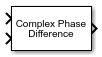# Complex Phase Difference

Phase difference between two complex signals

• Library:
• Communications Toolbox / Utility Blocks

•## Description

The Complex Phase Difference block computes the phase difference in radians between the second input signal and the first input signal. The elements of the output are between -π and π. This block independently processes each pair of corresponding elements.

## Ports

### Input

expand all

First input signal, specified as a scalar, column vector, or matrix. If both input signals are nonscalar, they must be the same dimension.

Note

This block processes complex signals. Real values at the input ports are type cast to complex values with `+j0` complex components.

Data Types: `double` | `single`
Complex Number Support: Yes

Second input signal, specified as a scalar, column vector, or matrix. If both input signals are nonscalar, they must be the same dimension. In2 must be the same data type as In1.

Note

This block processes complex signals. Real values at the input ports are type cast to complex values with `+j0` complex components.

Data Types: `double` | `single`

### Output

expand all

Phase shift difference in radians, returned as a scalar, column vector, or matrix. If either input signal is nonscalar, the output signal dimension matches the dimension of the nonscalar input signal. The output is the phase difference between the second input signal and the first input signal. The elements of the output signal are between -π and π and are the same data type as the input signals.

## Block Characteristics

 Data Types `double` | `single` Multidimensional Signals `no` Variable-Size Signals `no`